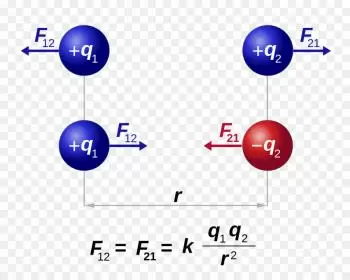# Coulomb's Law: Examples, Formula and LimitsCoulomb's law (or Coulomb's inverse-square law) defines the force exerted by an electric field on an electric charge. This is the force acting between electrically charged objects and is determined by the value of the interaction between two stationary point electric charges in a vacuum.

Coulomb's law states: "The electrical force of attraction or repulsion between two charges is inversely proportional to the square of the distance that separates them."

This force is called the Coulomb force and was described by the French physicist  Charles-Augustin de Coulomb in 1785. For this reason, the law was named after him and became the basis of electrostatics.

Coulomb's force is a consequence of Newton's third law that states that when two bodies interact, equal and opposite forces appear in each of them.

Charles-Augustin de Coulomb invented the torsion balance to measure magnetic and electrical attraction. This instrument can measure the torque resulting from the application of one or more forces to your arms.

## Coulomb's Law Formula

The following Coulomb's law formula allows to calculate the magnitude of the electric force between two charged particles:Where:

• F is the Coulomb force expressed in Newtons (N).

• 1 is the first point charge expressed in Coulombs (C).

• 2 is the second point charge (C).

• r is the distance between two point charges expressed in meters (m).

• C  is an electrostatic constant whose value is approximately 8.988 × 10 9 N · m 2 · C -2All the variables described in this definition correspond to the international system of units.

If the sign of the force is positive, it means that the force is repulsive. If, on the contrary, it is negative, the force is attractive.

From the formula, we can draw several conclusions that the force is directly proportional to the product of the electric charges q1 and q2

## What Are the Limitations of Coulomb's Law?

• The mathematical expression is only applicable to stationary point charges, and for more complicated static cases of charge, it needs to be generalized using the electric potential.

• When electric charges are in motion, it is necessary to replace even the Coulomb potential with the Liénard–Wiechert vector potential, especially if particle velocities are close to the speed of light.

• For charges at small distances (on the order of the size of atoms ), the effective electrostatic force must be corrected for quantum factors. However, for very intense fields, the spontaneous creation of particle-antiparticle pairs can occur, requiring updating the field for very short distances.

## What Are Electrostatic Forces?

Electrostatics is the branch of physics that studies the effects generated in bodies according to their electrical charges in equilibrium.

The electric force (F) is proportional to the charges brought together and is inversely proportional to the distance between them. This force acts between the charges radially, that is, a line between the charges; hence it is a radial vector between the two charges.

Likewise, this force depends on the electric charge and the distance between them. It is a fundamental principle of electrostatics and law applicable to charges at rest in a reference system.

It is worth mentioning that for small distances, the forces of the electric charges increase, and for considerable distances, the forces of the electric charges decrease. Hence, it is reduced as the charges move away from each other.

## The Magnitude of Coulomb's Force

The magnitude of the electromagnetic force is the one that affects the bodies that contain an electric charge, and that can lead to a physical or chemical transformation given that the bodies can attract or repel each other.

Therefore, the magnitude exerted on two electric charges is equal to the constant of the medium in which the electric charges are located by the quotient between the product of each of them and the distance that separates them squared.

The magnitude of the electrostatic force is proportional to the product of the magnitude of the charges q1 x q2. The electrostatic force at a short distance is mighty.

## Coulomb's Law Examples

Coulomb's law applies whenever electric charges and electric fields are involved. Some examples in everyday life are:

• An electromagnet: The force that an electromagnet generates when a current flows through it.

• Electric motors: the force of electric motors comes from the interaction of electrical charges.

• Atoms: atomic nuclei are made up of positive particles that exert a repulsive force. Nuclear bonds have to compensate for these forces.

• Balloon charged with electrostatic electricity: if we rub an air balloon with a woolen sweater, some electrons from the sweater pass into the balloon. If we place the balloon on the roof, it does not fall because the excess electrons (negatively charged) exert an attractive force on the protons (positively charged) of the atoms on the roof.

## Example Exercise of Using Coulomb's Law

Determine the electric force between two charged spheres of 1 μC each, placed at a distance of 1.0 cm.

Carrying out the exercise

Two electric charges are placed at a distance of 1 cm from each other.

We want to determine the electrical force between the two electrical charges, knowing that each charge has an intensity equal to 1 μC.

We apply Coulomb's law directly:

In which:

Q1 = Q2 = Q = 1 μC = 10 -6 C.

r = 1.0 cm = 10 -2 m

By replacing the formula we have:

F = 8.988 · 10 9 · (10 -6 · 10 -6 ) / 10 -2 = 89.88 N

Author:

Published: September 17, 2021
Last review: September 17, 2021